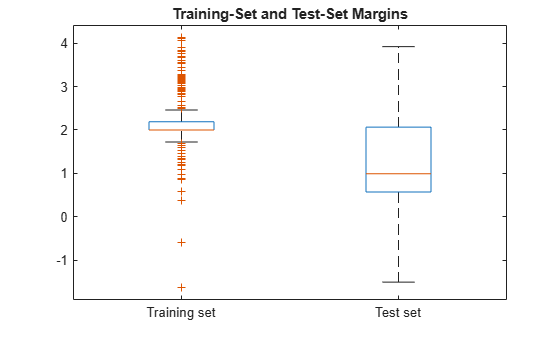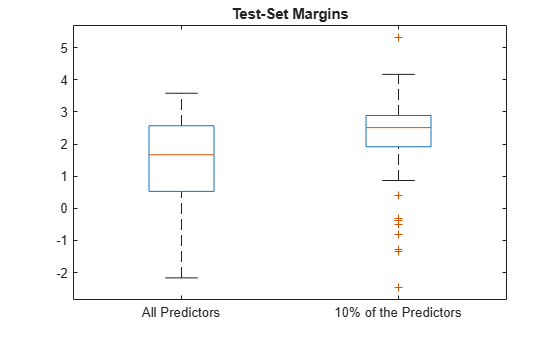# margin

Classification margins for Gaussian kernel classification model

## Syntax

``m = margin(Mdl,X,Y)``

## Description

example

````m = margin(Mdl,X,Y)` returns the classification margins for the binary Gaussian kernel classification model `Mdl` using the predictor data in `X` and the corresponding class labels in `Y`.```

## Examples

collapse all

Load the `ionosphere` data set. This data set has 34 predictors and 351 binary responses for radar returns, either bad (`'b'`) or good (`'g'`).

`load ionosphere`

Partition the data set into training and test sets. Specify a 30% holdout sample for the test set.

```rng('default') % For reproducibility Partition = cvpartition(Y,'Holdout',0.30); trainingInds = training(Partition); % Indices for the training set testInds = test(Partition); % Indices for the test set```

Train a binary kernel classification model using the training set.

`Mdl = fitckernel(X(trainingInds,:),Y(trainingInds));`

Estimate the training-set margins and test-set margins.

```mTrain = margin(Mdl,X(trainingInds,:),Y(trainingInds)); mTest = margin(Mdl,X(testInds,:),Y(testInds));```

Plot both sets of margins using box plots.

```boxplot([mTrain; mTest],[zeros(size(mTrain,1),1); ones(size(mTest,1),1)], ... 'Labels',{'Training set','Test set'}); title('Training-Set and Test-Set Margins')```The margin distribution of the training set is situated higher than the margin distribution of the test set.

Perform feature selection by comparing test-set margins from multiple models. Based solely on this criterion, the classifier with the larger margins is the better classifier.

Load the `ionosphere` data set. This data set has 34 predictors and 351 binary responses for radar returns, either bad (`'b'`) or good (`'g'`).

`load ionosphere`

Partition the data set into training and test sets. Specify a 15% holdout sample for the test set.

```rng('default') % For reproducibility Partition = cvpartition(Y,'Holdout',0.15); trainingInds = training(Partition); % Indices for the training set XTrain = X(trainingInds,:); YTrain = Y(trainingInds); testInds = test(Partition); % Indices for the test set XTest = X(testInds,:); YTest = Y(testInds);```

Randomly choose 10% of the predictor variables.

```p = size(X,2); % Number of predictors idxPart = randsample(p,ceil(0.1*p));```

Train two binary kernel classification models: one that uses all of the predictors, and one that uses the random 10%.

```Mdl = fitckernel(XTrain,YTrain); PMdl = fitckernel(XTrain(:,idxPart),YTrain);```

`Mdl` and `PMdl` are `ClassificationKernel` models.

Estimate the test-set margins for each classifier.

```fullMargins = margin(Mdl,XTest,YTest); partMargins = margin(PMdl,XTest(:,idxPart),YTest);```

Plot the distribution of the margin sets using box plots.

```boxplot([fullMargins partMargins], ... 'Labels',{'All Predictors','10% of the Predictors'}); title('Test-Set Margins')```The margin distribution of `PMdl` is situated higher than the margin distribution of `Mdl`. Therefore, the `PMdl` model is the better classifier.

## Input Arguments

collapse all

Binary kernel classification model, specified as a `ClassificationKernel` model object. You can create a `ClassificationKernel` model object using `fitckernel`.

Predictor data, specified as an n-by-p numeric matrix, where n is the number of observations and p is the number of predictors used to train `Mdl`.

The length of `Y` and the number of observations in `X` must be equal.

Data Types: `single` | `double`

Class labels, specified as a categorical, character, or string array, logical or numeric vector, or cell array of character vectors.

• The data type of `Y` must be the same as the data type of `Mdl.ClassNames`. (The software treats string arrays as cell arrays of character vectors.)

• The distinct classes in `Y` must be a subset of `Mdl.ClassNames`.

• If `Y` is a character array, then each element must correspond to one row of the array.

• The length of `Y` and the number of observations in `X` must be equal.

Data Types: `categorical` | `char` | `string` | `logical` | `single` | `double` | `cell`

## Output Arguments

collapse all

Classification margins, returned as an n-by-1 numeric column vector, where n is the number of observations in `X`.

collapse all

### Classification Margin

The classification margin for binary classification is, for each observation, the difference between the classification score for the true class and the classification score for the false class.

The software defines the classification margin for binary classification as

`$m=2yf\left(x\right).$`

x is an observation. If the true label of x is the positive class, then y is 1, and –1 otherwise. f(x) is the positive-class classification score for the observation x. The classification margin is commonly defined as m = yf(x).

If the margins are on the same scale, then they serve as a classification confidence measure. Among multiple classifiers, those that yield greater margins are better.

### Classification Score

For kernel classification models, the raw classification score for classifying the observation x, a row vector, into the positive class is defined by

`$f\left(x\right)=T\left(x\right)\beta +b.$`

• $T\left(·\right)$ is a transformation of an observation for feature expansion.

• β is the estimated column vector of coefficients.

• b is the estimated scalar bias.

The raw classification score for classifying x into the negative class is f(x). The software classifies observations into the class that yields a positive score.

If the kernel classification model consists of logistic regression learners, then the software applies the `'logit'` score transformation to the raw classification scores (see `ScoreTransform`).